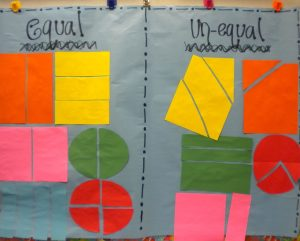# Fractions Part 2: Constructing and Drawing

by C. Elkins, OK Math and Reading LadyThe standards (CCSS or any state) use varied verbs to describe what students are to do regarding fractions: form, compose, construct, model, partition, draw, decompose, share, identify,  read, write, describe, order, and compare. Satisfying these standards can often be accomplished through use of concrete methods (manipulatives) and pictorial models (drawings). Remember the best understanding of concepts usually follows the concrete, pictorial, abstract progression (CPA). In other words, “Let’s make it, draw it, and then use numbers to represent it.”

Through constructing and drawing, students will  be prepared for further work with fractions, and they begin to conceptualize the relationship between the size of denominators, the numerators, and the whole. Click here for a FREE copy of the pictures you will see below (3 page pdf).

Form / Compose / Construct / Model:  Use smaller shapes to form or compose larger shapes (which is also a geometry std. in KG and 1st). Put together fraction pieces or puzzles to make the whole shape (circle, rectangle, hexagon, etc.). Use fraction pieces to demonstrate understanding by constructing models of area, set, and length.

These pictures show different ways students can use manipulatives to form, compose, construct, and model fractional parts (pattern blocks, fraction circles, tangrams, linking cubes, color tiles, fraction bars, Cuisenaire rods, two-color counters):Partition / Draw / Decompose / Share: Split larger shapes into smaller fractional parts (halves, thirds, fourths, etc.). Divide (fair share) objects into equal groups. Use models to decompose a fraction in more than one way. Represent fractions on a number line.

I enjoy teaching children how to partition common shapes into fractional parts – because it involves drawing. Too often, if I just tell them to divide a rectangle or circle into fourths or sixths, I get something like this: Continue reading

# Fractions Part I: Basics KG-2nd grade

by C. Elkins, OK Math and Reading LadyThis is the first post of several I will devote to fractions, starting with basic understanding in first grade and moving up toward operations with fractions in 5th and 6th grade. I would love to hear from you about your students successes and/or difficulties with fractions so I can be sure to address this topic to meet your needs. Free resources below.

What is a fraction?  A fraction represents a part of a whole. It consists of a numerator (which tells how many parts we are describing) and a denominator (how many parts the whole is divided into).

Some basics:

• Fractional parts must be equal. This is a concept introduced in first grade. (See some lesson plan ideas below.)From Pinterest

• The larger the denominator, the smaller the parts AND the smaller the denominator, the larger the parts — when comparing identical sized objects. You can’t compare 1/4 of a cookie with 1/4 of a cake. This is one of the hardest concepts to grasp – so lots of hands-on experience is needed.
• Helpful manipulatives to use with fractions: pattern blocks, color tiles, Cuisenaire rods, fraction circles, fraction strips, fraction bars, graham crackers.
• Be careful about always referring to fractions as “the shaded part.” While this might be true with pictures on worksheets, fractions can be described in these ways also: What fraction of the students are boys? What fraction of the pizza was eaten? What fraction of the candy bar is left? What fraction would belong here on the number line?
• Lines do not necessarily define the fractional part. On the picture shown, the left shows 1/4 shaded. The right also shows 1/4 shaded, but students are likely to say 1/3. Why? Because they count the parts shown (3) and the shaded parts (1) and put that together as a fraction. A way to show this is still 1/4 is to show that the shaded part will fit into the whole shape 4 times.• A unit fraction: This is a fraction with 1 as the numerator (1/4, 1/8, etc.). It is one unit of the whole.
• A fraction that is one unit away from a whole has a numerator one less than the denominator. Examples: 2/3, 3/4, 7/8, 11/12, etc. This is helpful to conceptualize when comparing fractions.
• When reading a fraction number line, compare it to a bar model. Then it is easier to see it is the spaces are the focus, not the tick marks.